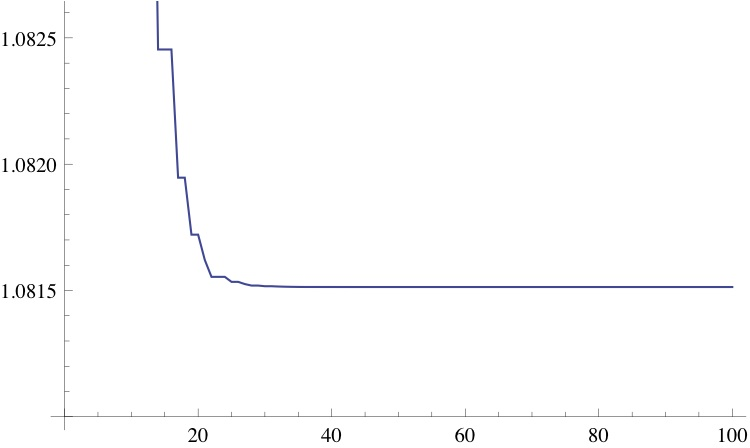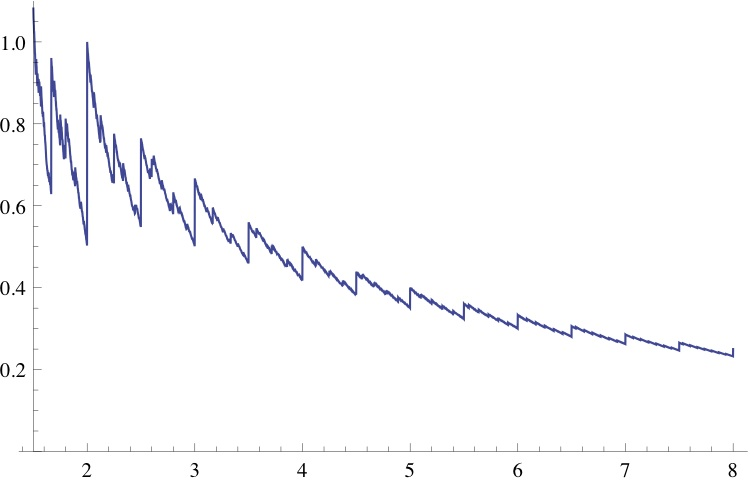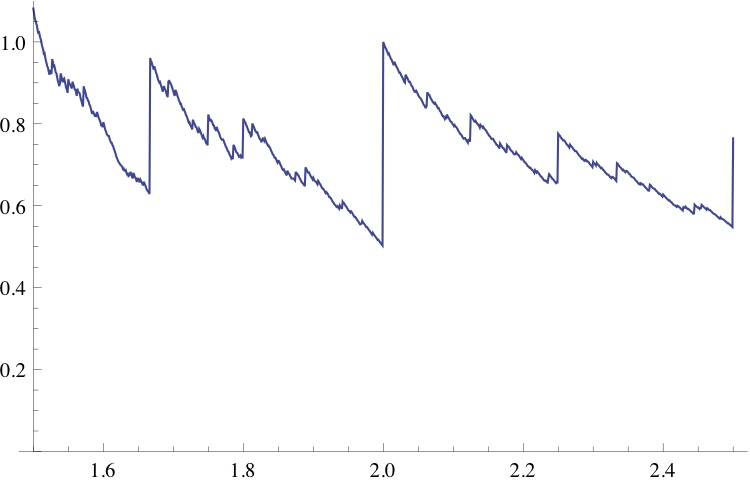# Growth rates of simple integer recurrences

Posted by: Gary Ernest Davis on: June 5, 2012

The sequence of integers$A(n)$ defined by$A(1)=2 \textrm{ and } A(n)= \lfloor(3/2)A(n-1)\rfloor$ – where$\lfloor x\rfloor$ , the floor of$x$ , is the greatest integer$\leq x$ – beginsÂ 2, 3, 4, 6, 9, 13, 19, 28, 42, 63, 94, 141, 211, 316, 474, 711, 1066, 1599, 2398, 3597, 5395, 8092, 12138, 18207, 27310, 40965, 61447, 92170, 138255, 207382, 311073 and grows rapidly.

One can numerically estimate the growth rate of this sequence by first observing that the sequence of positive numbers$\frac{A(n)}{(3/2)^n}$ is decreasing. This follows from the inequality$A(n) =\lfloor(3/2)A(n-1)\rfloor \leq (3/2)A(n-1)$ upon dividing by$(3/2)^n$, to get$\frac{A(n)}{(3/2)^n}\leq \frac{A(n-1)}{(3/2)^{n-1}}$.

This means the sequenceÂ$\frac{A(n)}{(3/2)^n}$ has a limit. In fact this sequence converges fairly rapidly to the limit:n A(n)/(3/2)n n A(n)/(3/2)n 1 1.33333333333 34 1.08151439525 2 1.33333333333 35 1.08151405187 3 1.18518518519 36 1.08151382295 4 1.18518518519 37 1.08151382295 5 1.18518518519 38 1.08151382295 6 1.14128943759 39 1.08151375512 7 1.11202560585 40 1.08151375512 8 1.09251638470 41 1.08151372497 9 1.09251638470 42 1.08151370487 10 1.09251638470 43 1.08151369148 11 1.08673587473 44 1.08151368254 12 1.08673587473 45 1.08151367659 13 1.08416675918 46 1.08151367262 14 1.08245401549 47 1.08151366997 15 1.08245401549 48 1.08151366997 16 1.08245401549 49 1.08151366880 17 1.08194653587 50 1.08151366880 18 1.08194653587 51 1.08151366880 19 1.08172098938 52 1.08151366880 20 1.08172098938 53 1.08151366880 21 1.08162074649 54 1.08151366880 22 1.08155391790 55 1.08151366869 23 1.08155391790 56 1.08151366862 24 1.08155391790 57 1.08151366862 25 1.08153411684 58 1.08151366862 26 1.08153411684 59 1.08151366860 27 1.08152531636 60 1.08151366860 28 1.08151944938 61 1.08151366859 29 1.08151944938 62 1.08151366859 30 1.08151684183 63 1.08151366859 31 1.08151684183 64 1.08151366859 32 1.08151568292 65 1.08151366859 33 1.08151491032 66 1.08151366859

x

So we see that$A(n)\approx 1.08151366859(3/2)^n$ for large$n$.

What if we replace the constant 3/2 by a parameter$r$ ?

How does the sequenceÂ$A(n)$ defined by$A(1)=2 \textrm{ and } A(n)= \lfloor rA(n-1)\rfloor$ grow?

As before we see there is a constant$K(r)$ such that$A(n)/r^n \to K(r) \textrm{ as } r \to \infty$

An obvious question is: how does$K(r) \textrm{ vary with } r$ ?

A moment’s thought shows that the answer is only interesting for$r \geq 3/2$

The following MathematicaÂ® code computes$K(r)$ to a decent approximation:

A[1, r_] = 2;
A[n_, r_] := A[n, r] = Floor[r*A[n – 1, r]];
T = Table[{r, Table[N[A[n, r]/r^n], {n, 1, 100}][[-1]]}, {r, 1.5, 5, 0.001}];
ListPlot[T, Joined -> True]

and the resulting plot of$K(r) \textrm{ versus } r$ looks as follows:A blow-up of the portion of the plot for$1.5 \leq r \leq 2.5$ looks as follows:### 1 Response to "Growth rates of simple integer recurrences"Some questions that came to my mind as I read this:

1. Is K(r) rational or irrational? Algebraic or transcendental?
2. How does the initial value of the sequence change the behavior of the sequence?

Something for me to play around with in my spare time. Thanks!